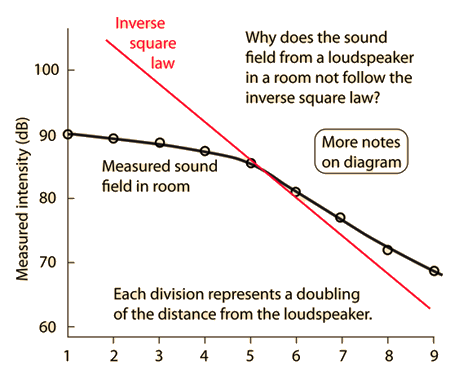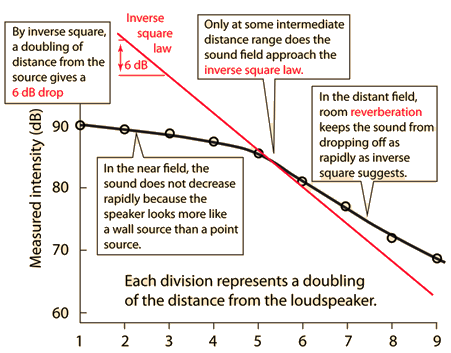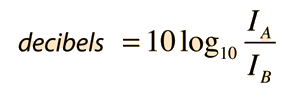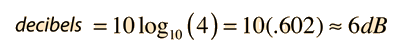# Measured Sound in a Room

 If the sound field in a room followed the inverse square law, then each doubling of distance from the source would give a drop of 6 decibels. The descending straight line on the plot at right represents inverse square law behavior, but you can see that the measured sound does not behave like that except in a narrow distance range.Effect of doubling distance
Index

Auditorium acoustics

 HyperPhysics***** Sound R Nave
Go Back

# Notes on Room Sound Measurement

Measurements in a laboratory room. Enclosed 6x9 inch speaker, white noise.

 # d(cm) dB dBA 1 2 90 90 2 4 89.6 89.4 3 8 88.8 88.8 4 16 87.1 87.7 5 32 86 85.4 6 64 81.5 80.6 7 128 77 76.4 8 256 72.2 71.6 9 512 68.8 68.3
The measurements in decibels and dBA were very similar, indicating that the room was fairly free of low frequency, inaudible sounds.Click on highlighted text for more detail.
 Description of measurement Inverse square law Reverberation Effect of doubling distance
Index

Auditorium acoustics

 HyperPhysics***** Sound R Nave
Go Back

# Effect of Doubling Distance

If the inverse square law applies, then doubling the distance from a point sound source will cause the intensity to drop by 6 decibels. The intensity difference in decibels between two sounds A and B is given byIf sound B is measured at double the distance of sound A from a point source so that it follows the inverse square law, then its intensity will be one fourth that of A. The difference in decibels is thenIn a real room, reverberation will contribute to the sound field so that the sound will not drop off as rapidly as the inverse square law predicts.

 Measurement for a real room Example for sound amplification system
Index

Auditorium acoustics

 HyperPhysics***** Sound R Nave
Go Back